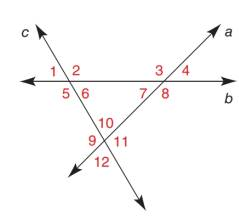Chapter 2.1, Problem 8EElementary Geometry For College St...

7th Edition
Alexander + 2 others
ISBN: 9781337614085

Solutions

Chapter
SectionElementary Geometry For College St...

7th Edition
Alexander + 2 others
ISBN: 9781337614085
Textbook Problem

Use drawings, as needed, to answer each question.With respect to c as a transversal for lines a and b,a) which angle corresponds to ∠ 5 ?b) which angle is the alternate interior angle for ∠ 2 ?Exercises 7, 8

To determine

a)

To find:

Angle corresponding to 5.

Explanation

Given:

The given figure is,

b is a transversal for lines a and c.

Definition:

Corresponding angles are created where a transversal intersects other lines. The corresponding angles are the ones at the same location at each intersection.

Approach:

Consider the given figure,

Figure (1)

From the figure (1), b is a transversal for lines a and c...

To determine

b)

To find:

Alternate interior angle for 2.

Still sussing out bartleby?

Check out a sample textbook solution.

See a sample solution

The Solution to Your Study Problems

Bartleby provides explanations to thousands of textbook problems written by our experts, many with advanced degrees!

Get Started

Evaluate the integrals in Problems 7-36. Check your results by differentiation. 22.

Mathematical Applications for the Management, Life, and Social Sciences

Find d9dx9(x8lnx).

Single Variable Calculus: Early Transcendentals, Volume I

In Exercises 9 and 10, find the limit. limx3(2x23x+4)

Calculus: An Applied Approach (MindTap Course List)

In Exercise 724, Compute the indicated products. 3

Finite Mathematics for the Managerial, Life, and Social Sciences

The vector represented by where A(4, 8) and B(6, 6)is:

Study Guide for Stewart's Multivariable Calculus, 8th